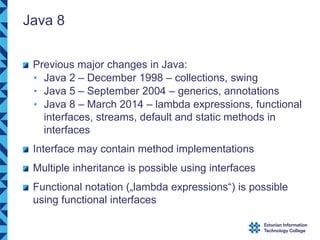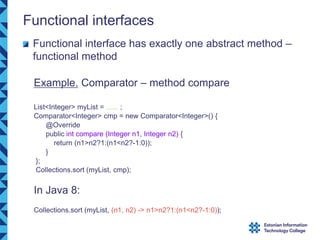Successfully reported this slideshow.

# documents.pub_new-features-in-java-8-it-jpoialjavanaitedwien15java8pdf-java-8-previous.pdf×

# documents.pub_new-features-in-java-8-it-jpoialjavanaitedwien15java8pdf-java-8-previous.pdf

This document presents all the new features introduces as a part of Java 8 version includes Lamdas.

This document presents all the new features introduces as a part of Java 8 version includes Lamdas.

### documents.pub_new-features-in-java-8-it-jpoialjavanaitedwien15java8pdf-java-8-previous.pdf

1. 1. New Features in Java 8 Jaanus Pöial, PhD Tallinn, Estonia
2. 2. Java 8 Previous major changes in Java: • Java 2 – December 1998 – collections, swing • Java 5 – September 2004 – generics, annotations • Java 8 – March 2014 – lambda expressions, functional interfaces, streams, default and static methods in interfaces Interface may contain method implementations Multiple inheritance is possible using interfaces Functional notation („lambda expressions“) is possible using functional interfaces
3. 3. Functional interfaces Functional interface has exactly one abstract method – functional method Example. Comparator – method compare List<Integer> myList = ...... ; Comparator<Integer> cmp = new Comparator<Integer>() { @Override public int compare (Integer n1, Integer n2) { return (n1>n2?1:(n1<n2?-1:0)); } }; Collections.sort (myList, cmp); In Java 8: Collections.sort (myList, (n1, n2) -> n1>n2?1:(n1<n2?-1:0));
4. 4. Lambda expressions Short way to express contents of functional interfaces param -> expression (param_1, ... , param_n) -> expression Class::method Class::new ... Expression can also be a block (in curly braces) Java.util.function.Function<Double, Double> fn; fn = x->x*(x-3.)*(x+4)*Math.cos(x); fn = Math::cos;
5. 5. User defined functional interface Example: @FunctionalInterface interface Talker<X> { void talk (X x); } public static void main (String[] args) { Talker<Integer> italk = i -> System.out.println ("int " + i); Talker<Double> dtalk = d -> System.out.println ("double " + d); italk.talk(45); dtalk.talk(Math.PI); }
6. 6. Streams Provide inner iteration for data structures (e.g. Stream for the elements of Collection) – parallel processing possible Packages involved: • java.lang – Iterable, ... • java.util – Optional, Collection, ... • java.util.stream – Stream, Collector, ... • java.util.function – Function,Predicate, Consumer, Supplier, BiFunction, ... Stream methods: map, filter, reduce, forEach, collect, flatMap, allMatch, max, min, distinct, generate, ... Optional methods: filter, map,flatMap, orElse, ifPresent, ...
7. 7. Example - outer iteration // Old way (Java 1) sum = 0; for (int i = 0; i < myList.size(); i++) { if (myList.get(i) > 0) { sum += myList.get (i); } } // little better old way (Java 5) sum = 0; for (int elem : myList) { if (elem > 0) { sum += elem; } }
8. 8. Stream example - Consumer // forEach and anonymous Consumer (ugly) sum = 0; myList.forEach (new Consumer<Integer>() { @Override public void accept (Integer elem) { if (elem > 0) { sum += elem; } } });
9. 9. Stream example - lambda // Java 8 forEach and lambda expression sum = 0; myList.forEach (elem -> { if (elem > 0) { sum += elem; } }); System.out.println ("SumPos is: " + sum);
10. 10. Stream example – filter and reduce // Java 8 stream, filter, reduce (with lambda expression) - the best sum = myList.stream() .filter (elem -> (elem > 0)) .reduce (0, (s, e) -> s + e);
11. 11. Stream example – map and optional // multiply each element of the list by 2 and find the first element // that is bigger than 3 (null, if there is no such element) myList.stream() .map (e->e*2) .filter (e->(e>3)) .findFirst() .orElse (null)
12. 12. Example - user defined map public static void main (String[] args) { "Hello World".chars() .map (J8example5::myMap) .forEach (ch -> System.out.print ((char)ch) ); System.out.println(); } public static int myMap (int chi) { char ch = (char)chi; if (Character.isLowerCase(ch)) return Character.toUpperCase(ch); else if (Character.isUpperCase(ch)) return Character.toLowerCase(ch); else return ch; }
13. 13. // Map as expression public static void main (String[] args) { "Hello World 2015".chars() .map (ch -> Character.isLowerCase (ch)? Character.toUpperCase (ch): (Character.isUpperCase (ch)? Character.toLowerCase (ch): ch) ) .forEach (ch -> System.out.print ((char)ch)); System.out.println(); }
14. 14. Adding method implementations to interfaces Default methods in interface provide implementation, if it is not provided by the class. Overriding is OK. Static methods in interface provide implementation that can be used in default methods (or elsewhere). Overriding is not OK. Methods defined in class are always „stronger“ than methods defined in interface. If a class implements two (or more) interfaces that have the same method, it is up to the class to decide about implementation of this method (diamond problem).
15. 15. Example of default and static methods // talk must be overriden, log can be overriden, newlog can be used @FunctionalInterface interface Talker<X> { void talk (X x); // compulsory method default void log (X x) { // possible to override System.out.println ("logged by log in Talker interface: " + x); newlog (x.toString()); } static void newlog (String s) { // impossible to override, possible to use System.out.println ("logged by newlog in Talker interface: " + s); } }
16. 16. // Class provides both talk and log static class MyTalker1<X> implements Talker<X> { @Override public void talk (X x) { System.out.println ("talk from MyTalker1: " + x); } @Override public void log (X x) { System.out.println ("logged by log in Mytalker1: " + x); System.out.println ("also call to newlog by log in MyTalker1:"); Talker.newlog (x.toString()); // it is possible to use interface static method in class } }
17. 17. // Class does not provide log static class MyTalker2<X> implements Talker<X> { @Override public void talk (X x) { System.out.println ("talk from MyTalker2: " + x); } } // test public static void main (String[] args) { Talker<Integer> italk = i -> System.out.println ("int " + i); Talker<Double> dtalk = d -> System.out.println ("double " + d); italk.talk(45); // int 45 dtalk.talk(Math.PI); // double pi MyTalker1<Integer> myitalk1 = new MyTalker1<Integer>(); myitalk1.talk (2014); // from class myitalk1.log (1022); // log from class contains static newlog from interface MyTalker2<Integer> myitalk2 = new MyTalker2<Integer>(); myitalk2.talk (2015); // from class myitalk2.log (1023); // from interface contains static newlog }
18. 18. Example about extending the interface @FunctionalInterface interface MyComparable<T> extends Comparable<T> { default boolean myEquals (T o2) { return this.compareTo (o2) == 0; } } static class MyInt implements MyComparable<MyInt> { private int content = 0; MyInt (int i) { content = i; } @Override public int compareTo (MyInt o) { // delegation to Integer return new Integer(content).compareTo (o.content); } @Override public boolean equals (Object o) { // override equals in class using interface return myEquals ((MyInt)o); } }
19. 19. Multiple inheritance using interfaces Multiple inheritance – ability to inherit behaviour from several superclasses Diamond Example. Vehicle Boat Amphibian Car Amphibian Boat Car Vehicle Amphibian
20. 20. @FunctionalInterface interface Vehicle { void makeNoise(); default void startEngine() { System.out.println ("Vehicle engine started"); } default void stopEngine() { System.out.println ("Vehicle engine stopped"); } }
21. 21. @FunctionalInterface interface Car extends Vehicle { // obligation to provide makeNoise @Override default void stopEngine() { System.out.println ("Car engine stopped"); } default void enjoyCar() { System.out.println ("I enjoy my car: Car interface default"); } default void drive() { System.out.println ("I drive my car: Car interface default"); } // possible to override also startEngine }
22. 22. @FunctionalInterface interface Boat extends Vehicle { // obligation to provide makeNoise default void enjoyBoat() { System.out.println ("I enjoy my boat: Boat interface default"); } default void drive() { System.out.println ("I drive my boat: Boat interface default"); } // possible to override also startEngine, stopEngine }
23. 23. static class Amphibian implements Car, Boat { @Override public void makeNoise() { // my obligation from Vehicle System.out.println ("makeNoise: compulsory Vehicle behaviour: from Amphibian class"); } @Override public void drive() { // diamond problem solved in Java way System.out.println ("I drive my amphibian: from Amphibian class"); } // possible to override also startEngine, stopEngine, enjoyCar, enjoyBoat }
24. 24. public static void main (String[] args) { Amphibian a = new Amphibian(); a.startEngine(); // Vehicle engine started a.makeNoise(); // both Car and Boat are kind of Vehicle a.drive(); // diamond problem (both Car and Boat provide drive method) a.enjoyCar(); // inherited from Car a.enjoyBoat(); // inherited from Boat a.stopEngine(); // Car is more specific than Vehicle } Vehicle engine started makeNoise: compulsory Vehicle behaviour: from Amphibian class I drive my amphibian: from Amphibian class I enjoy my car: Car interface default I enjoy my boat: Boat interface default Car engine stopped
25. 25. Playing with functions Functional interfaces are supported in java.util.function package Function<Double, Double> f = Math::sin; // assignment context: Class::method double res = f.apply (Math.PI/2.); // variable f represents a function System.out.println (res); // degrees  radians  sin : g takes degrees as argument and returns sinus Function<Double, Double> g = ((Function<Double, Double>)Math::sin) .compose (Math::toRadians); System.out.println (g.apply (45.)); ((Consumer<String>)System.out::println).accept (String.valueOf (g.apply (45.)));
26. 26. Functions on functions public static <T, U> Function<U, U> proj1 (BiFunction<T, U, U> b, T arg) { System.out.println ("(" + b + " in proj1 applied to " + arg); return y -> b.apply (arg, y); } public static <T, U> Function<T, T> proj2 (BiFunction<T, U, T> b, U arg) { System.out.println ("(" + b + " in proj2 applied to " + arg); return x -> b.apply (x, arg); } public static <T, U> Function<T, U> combine (Function<U, U> f, Function<T, U> g) { return x -> f.apply (g.apply (x)); }
27. 27. public static int minus (int a1, int a2) { System.out.println ("("+a1+"-"+a2+") "); return a1-a2; } BiFunction<Integer, Integer, Integer> p = J8example9::minus; Function<Integer, Integer> p1 = proj1 (p, 2); // p1(x) = 2 - x System.out.println (p1.apply (8)); // 2 – 8 = -6 Function<Integer, Integer> p2 = proj2 (p, 1); // p2(x) = x - 1 System.out.println (p2.apply (9)); // 9 – 1 = 8 System.out.println (combine (p1, p2).apply (4)); // ([2-x][x-1])(4) = [2-x]([x-1](4)) = [2-x](3) = 2-3 = -1
28. 28. public static <T, U, V> Function<T, V> sCombine (BiFunction<T, U, V> f, Function<T, U> g) { return x -> f.apply (x, g.apply (x)); } public static <T, U, V> BiFunction<T, U, V> sBiCombine (BiFunction<T, U, V> f, BiFunction<T, U, U> g) { return (x, y) -> f.apply (x, g.apply (x, y)); } BiFunction<Integer, Integer, Integer> p = J8example9::minus; BiFunction<Integer, Integer, Integer> q = Math::max; Function<Integer, Integer> p1 = proj1 (p, 2); // p1(x) = 2 - x System.out.println (sCombine (p, p1).apply (11)); // [x – [2-x] ](11) = 11-(2-11) = 20 System.out.println (sBiCombine (p, q).apply (17, 29)); // [x – max(x,y)](17,29) = 17-29 = -12 // sBiCombine (J8example9::sBiCombine, J8example9::sBiCombine).apply (17, 29); // does not compile
29. 29. Java FX became a part of Java 8 Example – drawing a graph of a function Class DrawFunction
30. 30. @Override public void start (Stage myStage) { // compulsory method for Java FX Application Function<Double, Double> fn = x->x*(x-3.)*(x+4)*Math.cos(x); double from = -5.; double to = 5.; Pane myPane = new Pane(); Scene myScene = new Scene (myPane, 319, 159); myStage.setScene (myScene); myStage.setTitle ("Graph of a function"); ObservableList<Node> nodes = myPane.getChildren(); drawFunction (fn, from, to, myScene.getWidth(), myScene.getHeight(), nodes); myScene.widthProperty().addListener ( (obsv, oldv, newv) -> { drawFunction (fn, from, to, myScene.getWidth(), myScene.getHeight(), nodes); }); myScene.heightProperty().addListener ( (obsv, oldv, newv) -> { drawFunction (fn, from, to, myScene.getWidth(), myScene.getHeight(), nodes); }); myStage.show(); }
31. 31. public static void drawFunction (Function<Double,Double> f, double start, double end, double w, double h, ObservableList<Node> nl) { int iw = (int)w; Double[] points = new Double[2*iw]; double fmax = Double.MIN_VALUE; double fmin = Double.MAX_VALUE; for (int i=0; i < iw; i++) { double arg = start + ((double)i)*(end-start)/w; double value = f.apply (arg); points[2*i] = (double)i; points[2*i+1] = value; // to be scaled later if (value > fmax) fmax = value; if (value < fmin) fmin = value; } for (int i=0; i < iw; i++) { double value = points[2*i+1]; points[2*i+1] = (fmax-value)*h/(fmax-fmin); // scaling } Polyline graph = new Polyline(); graph.getPoints().addAll (points); nl.clear(); nl.add (graph); }
32. 32. Exercises Instructions are given during the lectures